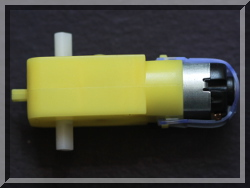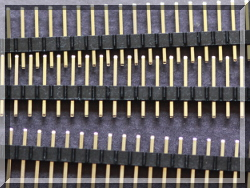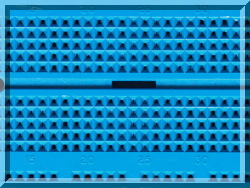lg lgpio C lgpio Python Daemon rgpio C rgpio Python rgs Download Examples FAQ Site Map

# Scripts

## Scripts

Scripts are programs to be stored and executed by the rgpiod daemon. They are intended to mitigate any performance problems associated with the daemon server/client model.

Scripts are work in progress.

### Virtual machine

A script runs within a virtual machine with

a 32 bit accumulator A.
a flags register F.
a program counter PC.

Each script has

10 parameters named 0 through 9.
150 variables named 0 through 149.
50 labels which are named by any unique number.

### Commands

Many lg commands may be used within a script. However some commands do not work within the script model as designed and are not permitted.

The following commands are not permitted within a script:

File - FL FO FR FW

I2C - I2CPK I2CRD I2CRI I2CRK I2CWD I2CWI I2CWK I2CZ

Script control - PARSE PROC PROCD PROCP PROCR PROCS PROCU

Serial - SERO SERR SERW SLR

SPI - SPIR SPIW SPIX

The following commands are only permitted within a script:

 Command Description Definition ADD x Add x to accumulator A+=x; F=A AND x And x with accumulator A&=x; F=A CALL L Call subroutine at tag L push(PC+1); PC=L CMP x Compare x with accumulator F=A-x DCR y Decrement register --*y; F=*y DCRA Decrement accumulator --A; F=A DIV x Divide x into accumulator A/=x; F=A HALT Halt Halt INR y Increment register ++*y; F=*y INRA Increment accumulator ++A; F=A JGE L Jump if >= 0 to tag L if (F>=0) PC=L JGT L Jump if > 0 to tag L if (F>0) PC=L JLE L Jump if <= 0 to tag L if (F<=0) PC=L JLT L Jump if < 0 to tag L if (F<0) PC=L JMP L Jump to tag L PC=L JNZ L Jump if non-zero to tag L if (F) PC=L JZ L Jump if zero to tag L if (!F) PC=L LD y x Load register with x *y=x LDA x Load accumulator with x A=x MLT x Multiply x with accumulator A*=x; F=A MOD x Modulus x with accumulator A%=x; F=A OR x Or x with accumulator A|=x; F=A POP y Pop register y=pop() POPA Pop accumulator A=pop() PUSH y Push register push(y) PUSHA Push accumulator push(A) RET Return from subroutine PC=pop() RL y x Rotate left register x bits *y<<=x; F=*y RLA x Rotate left accumulator x bits A<<=x; F=A RR y x Rotate right register x bits *y>>=x; F=*y RRA x Rotate right accumulator x bits A>>=x; F=A SHL y x Shift left register x bits *y<<=x; F=*y SHLA x Shift left accumulator x bits A<<=x; F=A SHR y x Shift right register x bits *y>>=x; F=*y SHRA x Shift right accumulator x bits A>>=x; F=A STA y Store accumulator in register y=A SUB x Subtract x from accumulator A-=x; F=A SYS str Run external script system(str); F=A TAG L Label the current position N/A X y1 y2 Exchange registers y1 and y2 t=*y1;*y1=*y2;*y2=t XA y Exchange accumulator and register t=A;A=*y;*y=t XOR x Xor x with accumulator A^=x; F=A

x may be a constant, a parameter (p0-p9), or a variable (v0-v149).

y may be a parameter (p0-p9), or a variable (v0-v149). If p or v isn't specified y is assumed to be a variable.

The SYS script receives two unsigned parameters: the accumulator A and the current GPIO levels.